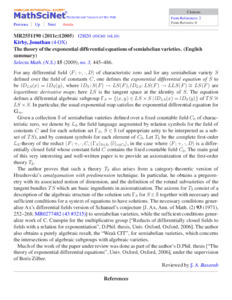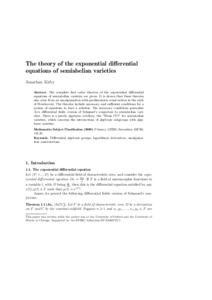# The theory of the exponential differential equations of semiabelian varieties

Kirby, J (2009) The theory of the exponential differential equations of semiabelian varieties. Selecta Mathematica, 15 (3). pp. 445-486. ISSN 1022-1824Preview
PDF (The_theory_of_the_exponential_differential.pdf)Preview
PDF (tedesv_selecta) - Accepted VersionView Item